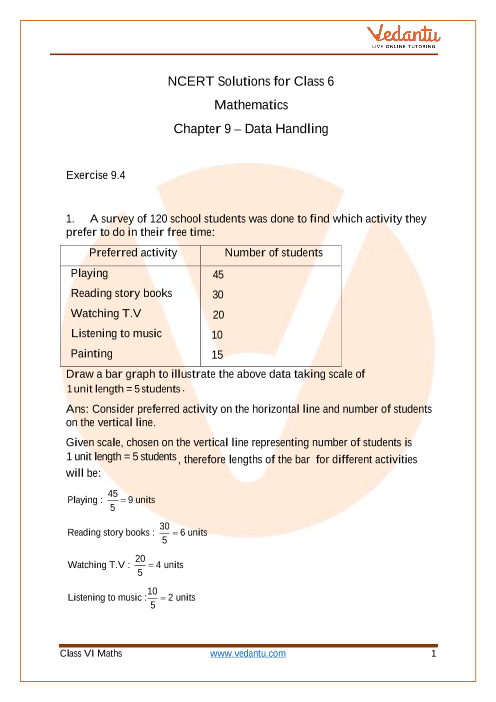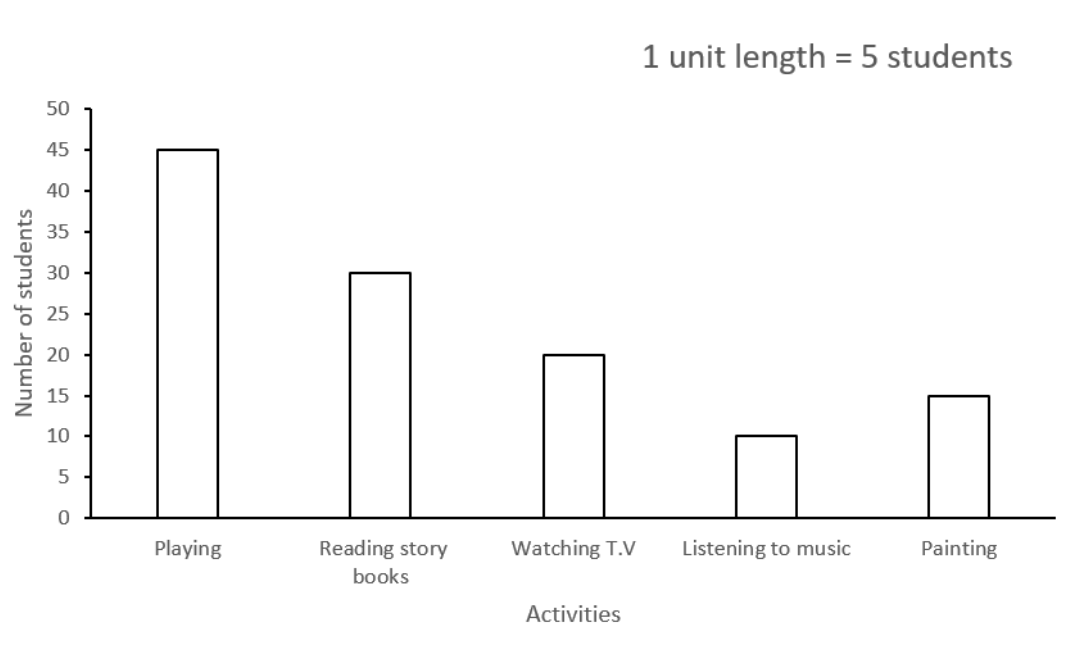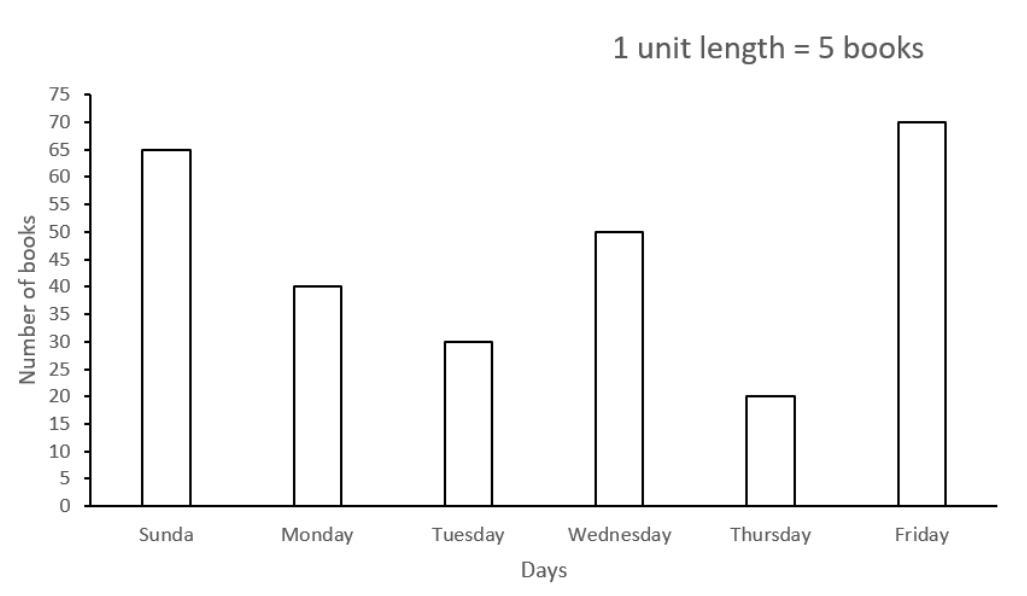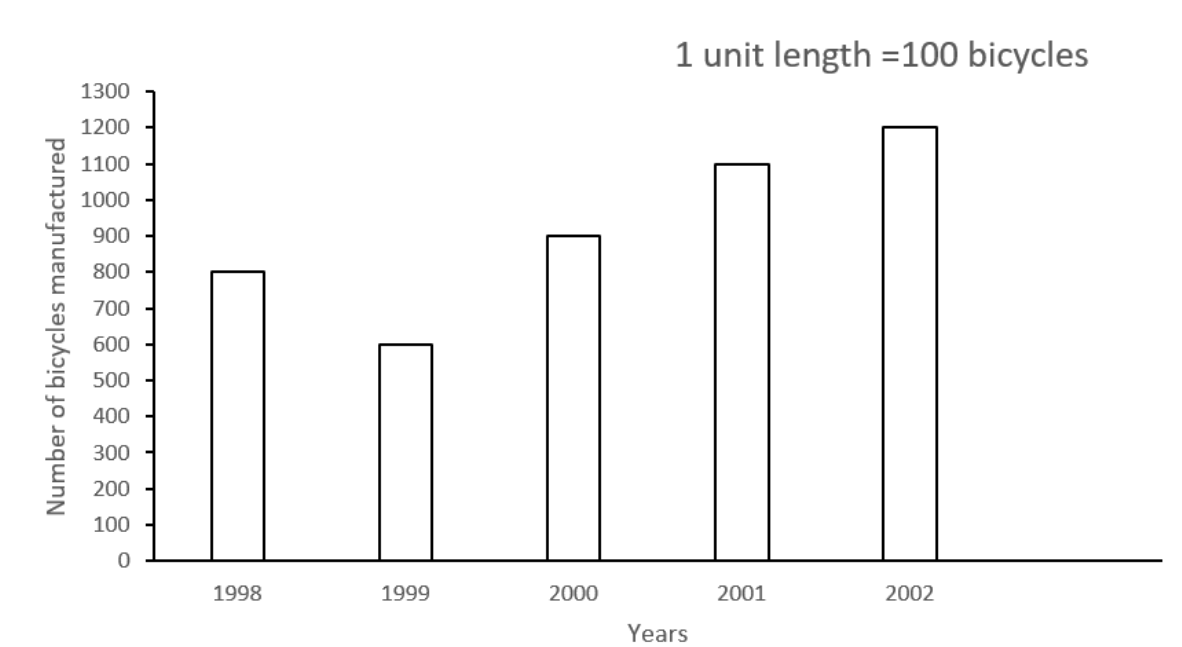# NCERT Solutions for Class 6 Maths Chapter 9 Data Handling (Ex 9.4) Exercise 9.4

## NCERT Solutions for Class 6 Maths Chapter 9 Data Handling (Ex 9.4) Exercise 9.4

Free PDF download of NCERT Solutions for Class 6 Maths Chapter 9 Exercise 9.4 (Ex 9.4) and all chapter exercises at one place prepared by expert teacher as per NCERT (CBSE) books guidelines. Class 6 Maths Chapter 9 Data Handling Exercise 9.4 Questions with Solutions to help you to revise complete Syllabus and Score More marks. Register and get all exercise solutions in your emails.

Do you need help with your Homework? Are you preparing for Exams?
Study without Internet (Offline)Book your Free Demo session
Get a flavour of LIVE classes here at Vedantu## Access NCERT Solutions for Class 6 Mathematics Chapter 9 – Data Handling

Exercise 9.4

1. A survey of $120$ school students was done to find which activity they prefer to do in their free time:

 Preferred Activity Number of Students Playing $45$ Reading Story Books $30$ Watching T.V $20$ Listening to Music $10$ Listening to Music ${\text{15}}$

Draw a bar graph to illustrate the above data taking scale of ${\text{1 unit length = 5 students}}$.

Ans: Consider preferred activity on the horizontal line and the number of students on the vertical line.

Given scale, chosen on the vertical line representing number of students is ${\text{1 unit length = 5 students}}$, therefore lengths of the bar  for different activities will be:

${\text{Playing : }}\dfrac{{45}}{5} = {\text{9 units}}$

${\text{Reading story books : }}\dfrac{{30}}{5} = {\text{6 units}}$

${\text{Watching T}}{\text{.V : }}\dfrac{{20}}{5} = 4{\text{ units}}$

${\text{Listening to music :}}\dfrac{{10}}{5} = 2{\text{ units}}$

${\text{Painting :}}\dfrac{{15}}{5} = 3{\text{ units}}$

Therefore, the bar graph will be:Which activity is preferred by most of the students other than playing?

Ans: Activity with a bar of length smaller than the bar of playing but greater than any other activity bar is the most preferred activity other than playing. Reading storybook is an activity with the bar of length smaller than the playing bar and greater than the bars of other activities. Therefore, reading a storybook is the most preferred activity by most of the students other than playing.

2. The number of mathematics books sold by a shopkeeper on six consecutive days is given below:

 Days Sunday Monday Tuesday Wednesday Thursday Friday Number of Books Sold $65$ $40$ $30$ $50$ $20$ $70$

Draw a graph to represent above information choosing the scale of your choice.

Ans: Consider days of the week on the horizontal line and number of books sold on the vertical line.

Let scale, chosen on the vertical line representing number of books sold be,${\text{1 unit length}} = 5{\text{ books}}$

Because scale, chosen on the vertical line representing number of books sold is ${\text{1 unit length}} = 5{\text{ books}}$, therefore lengths of the bar  for different days of the week  will be:

${\text{Sunday : }}\dfrac{{65}}{5} = 13{\text{ units}}$

${\text{Monday :}}\dfrac{{40}}{5} = 8{\text{ units}}$

${\text{Tuesday :}}\dfrac{{30}}{5} = 6{\text{ units}}$

${\text{Wednesday :}}\dfrac{{50}}{5} = 10{\text{ units}}$

${\text{Thursday :}}\dfrac{{20}}{5} = 4{\text{ units}}$

${\text{Friday :}}\dfrac{{70}}{5} = 14{\text{ units}}$

Therefore, the bar graph will be:3. Following table shows the number of bicycles manufactured in a factory during the years $1998$ to ${\text{2002}}$. Illustrate this data using a bar graph. Choose a scale of your choice.

 Years Number Of Bicycles Manufactured $1998$$1999$${\text{2000}}$${\text{2001}}$${\text{2002}}$ ${\text{800}}$${\text{600}}$${\text{900}}$${\text{1100}}$${\text{1200}}$

Ans: Consider years on the horizontal line and number of bicycles manufactured on the vertical line.

Let scale, chosen on the vertical line representing number of bicycles manufactured be${\text{1 unit length = 100 bicycles}}$.

Because scale, chosen on the vertical line representing number of bicycles manufactured sold is ${\text{1 unit length }} = {\text{ 100 bicycles}}$, therefore heights of the bar  for different years  will be:

${\text{1998 : }}\dfrac{{800}}{{100}} = 8{\text{ units}}$

${\text{1999 : }}\dfrac{{600}}{{100}} = 6{\text{ units}}$

${\text{2000 : }}\dfrac{{900}}{{100}} = 9{\text{ units}}$

${\text{2001 : }}\dfrac{{1100}}{{100}} = 11{\text{ units}}$

${\text{2002 : }}\dfrac{{1200}}{{100}} = 12{\text{ units}}$

Therefore, the bar graph will be:(a) In which year were the maximum number of bicycles manufactured?

Ans: Year with the highest bar is the year in which the maximum number of bicycles were manufactured. In the bar graph  ${\text{2002}}$ is the year with the highest bar, therefore ${\text{2002}}$ is the year in which a maximum number of bicycles were manufactured.

(b) In which year were the minimum number of bicycles manufactured?

Ans: Year with the smallest bar is the year in which a minimum number of bicycles were manufactured. In the bar graph  ${\text{1999}}$ is the year with the smallest bar, therefore ${\text{1999}}$ is the year in which a minimum number of bicycles were manufactured.

4. Number of persons in various age groups in a town is given in the following table.

 Age Groups(In Years) 1 - 14 15 - 29 30 - 44 45 - 59 60 - 74 75 &  above Number Of Persons 2 lakhs 1 lakh 60 thousand 1 lakh 20 thousand 1 lakh 20 thousand 80 thousand 40 thousand

Draw a bar graph to represent the above information and answer the following questions. (take${\text{1 unit length = 20 thousand persons}}$)

Ans: Consider age groups on the horizontal line and the number of persons on the vertical line.

Scale, chosen on the vertical line representing a number of persons is ${\text{1 unit length = 20 thousand persons}}$.

Because scale, chosen on the vertical line representing number of persons is ${\text{1 unit length = 20 thousand persons}}$, therefore lengths of the bar  for different age groups  will be:

${\text{1 - 14 : }}\dfrac{{2,00,000}}{{20,000}} = 10{\text{ units}}$

${\text{15 - 29 : }}\dfrac{{1,60,000}}{{20,000}} = 8{\text{ units}}$

${\text{30 - 44 : }}\dfrac{{1,20,000}}{{20,000}} = 6{\text{ units}}$

${\text{45 - 59 : }}\dfrac{{1,20,000}}{{20,000}} = 6{\text{ units}}$

${\text{60 - 74 : }}\dfrac{{80,000}}{{20,000}} = 4{\text{ units}}$

${\text{75 \& above : }}\dfrac{{60,000}}{{20,000}} = 3{\text{ units}}$

Therefore, the bar graph will be:

(a) Which two age groups have same population?

Ans: Age groups with equal length of bars are the age groups with the same population. $30 - 44$ and $45 - 59$ are age groups with equal length of bars (${\text{6 units}}$).Therefore age groups $30 - 44$ and $45 - 59$ are age groups with the same population.

(b) All persons in the age group of 60 and above are called senior citizens. How many senior citizens are there in the town?

Ans: ${\text{Number of senior citizens}} = {\text{ Number of persons in the age group }}60 - 74 + {\text{ Number of persons in the age group }}75{\text{ \& above}}$

Therefore,${\text{Number of senior citizens}} = 80,000 + 40,000$

${\text{Number of senior citizens}} = 1,20,000$

Hence, there are $1{\text{ lakh 20 thousand}}\quad$ senior citizens in the town.

## NCERT Solutions for Class 6 Maths Chapter 9 Data Handling Exercise 9.4

Opting for the NCERT solutions for Ex 9.4 Class 6 Maths is considered as the best option for the CBSE students when it comes to exam preparation. This chapter consists of many exercises. Out of which we have provided the Exercise 9.4 Class 6 Maths NCERT solutions on this page in PDF format. You can download this solution as per your convenience or you can study it directly from our website/ app online.

Vedantu in-house subject matter experts have solved the problems/ questions from the exercise with the utmost care and by following all the guidelines by CBSE. Class 6 students who are thorough with all the concepts from the Subject Maths textbook and quite well-versed with all the problems from the exercises given in it, then any student can easily score the highest possible marks in the final exam. With the help of this Class 6 Maths Chapter 9 Exercise 9.4 solutions, students can easily understand the pattern of questions that can be asked in the exam from this chapter and also learn the marks weightage of the chapter. So that they can prepare themselves accordingly for the final exam.

Besides these NCERT solutions for Class 6 Maths Chapter 9 Exercise 9.4, there are plenty of exercises in this chapter which contain innumerable questions as well. All these questions are solved/answered by our in-house subject experts as mentioned earlier. Hence all of these are bound to be of superior quality and anyone can refer to these during the time of exam preparation. In order to score the best possible marks in the class, it is really important to understand all the concepts of the textbooks and solve the problems from the exercises given next to it.

Do not delay any more. Download the NCERT solutions for Class 6 Maths Chapter 9 Exercise 9.4 from Vedantu website now for better exam preparation. If you have the Vedantu app in your phone, you can download the same through the app as well. The best part of these solutions is these can be accessed both online and offline as well.SHARETWEETSHARESUBSCRIBE